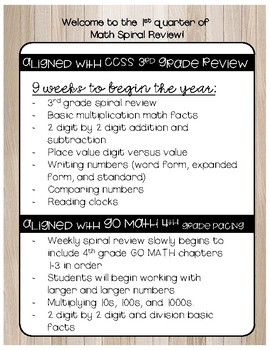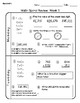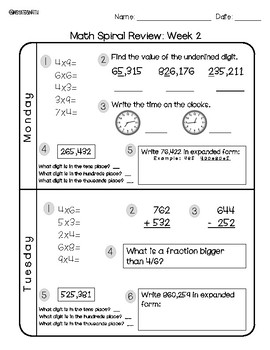# CCSS AND Go Math Aligned Spiral Review to START the Year With!Subject
Resource Type
File Type

Zip

(11 MB|40 pages)
Standards
• Product Description
• Standards

1st Quarter Spiral Review! Now including an EDITABLE version!

How do you begin spiral reviewing RIGHT off the bat?? You use previously taught skills to your advantage, so when you plan your mini-lessons you know that you can build on those 3rd grade standards!

9 weeks worth of Monday through Thursday spiral review::

- Basic multiplication math facts

- 2 digit by 2 digit addition and subtraction

- Place value digit versus value

- Writing numbers (word form, expanded form, and standard)

- Comparing numbers

- Elapsed Time

- Basic fractions

- Rounding to the hundreds place

Aligned with GO MATH 4th Grade Scope and Sequence!

- Weekly spiral review slowly begins to include 4th grade GO MATH chapters 1-3 in order

- Students will begin working with larger and larger numbers for place value concepts, adding/subtraction, expanded form, rounding, etc.

- Multiplying 10s, 100s, and 1000s.

- 2 digit by 2 digit

- Division basic facts

- Dividing 10s, 100s, and 1000s.

- Rounding to the hundred thousands place

I use this by having it in my student’s ”work in progress” folder to complete during any free time, when they finish something early, are waiting during a transition, etc. since it is an independent review of previously taught skills.

IF YOU SHARE YOUR PURCHASE ON INSTAGRAM AND TAG @MSYATESIN4TH IN IT, I WILL SEND YOU A FREE ITEM FROM MY STORE OF YOUR CHOICE!

Partition shapes into parts with equal areas. Express the area of each part as a unit fraction of the whole. For example, partition a shape into 4 parts with equal area, and describe the area of each part as 1/4 of the area of the shape.
Represent a fraction 1/𝘣 on a number line diagram by defining the interval from 0 to 1 as the whole and partitioning it into 𝘣 equal parts. Recognize that each part has size 1/𝘣 and that the endpoint of the part based at 0 locates the number 1/𝘣 on the number line.
Understand a fraction as a number on the number line; represent fractions on a number line diagram.
Interpret a multiplication equation as a comparison, e.g., interpret 35 = 5 × 7 as a statement that 35 is 5 times as many as 7 and 7 times as many as 5. Represent verbal statements of multiplicative comparisons as multiplication equations.
Fluently multiply and divide within 100, using strategies such as the relationship between multiplication and division (e.g., knowing that 8 × 5 = 40, one knows 40 ÷ 5 = 8) or properties of operations. By the end of Grade 3, know from memory all products of two one-digit numbers.
Total Pages
40 pages
Included
Teaching Duration
N/A
Report this Resource to TpT
Reported resources will be reviewed by our team. Report this resource to let us know if this resource violates TpT’s content guidelines.